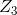# 14. Extra Credit: Cayley’s Theorem is an important one in advanced algebra. It says that “Every...

14. Extra Credit: Cayley’s Theorem is an important one in advanced algebra. It says that “Every algebraic group is isomorphic to some permutation group.” Demonstrate this to be true by finding a permutation group (Sn, ∘ ) that is isomorphic to (ℤ3, +) for some n.

## Solutions

##### Expert Solutionwe have verified cayleys theorem for the group.We have found an isomorphism to group of even permutations.so please see thenote above to get a better understanding.

## Related Solutions

##### Linear algebra Thank you. Extra Credit (No partial credit - 1%): It is often useful to...
Linear algebra Thank you. Extra Credit (No partial credit - 1%): It is often useful to perform a change of basis to examine an object from a different perspective. In the process of a larger work the conic  work the conic 3 x square − 10 x y + 3 y square + 16 x root 2 − 32 = 0 needs to be identified; this can be accomplished by a rotation. Identify the given conic and give its equation in...
##### Central limit theorem is important because? For a large , it says the population is approximately...
Central limit theorem is important because? For a large , it says the population is approximately normal. For a large , it says the sampling distribution of the sample mean is approximately normal, regardless of the shape of the population. For any population, it says the sampling distribution of the sample mean is approximately normal, regardless of the sample size. For any sized sample, it says the sampling distribution of the sample mean is approximately normal.
##### According to the Fundamental Theorem of Algebra, every nonconstant polynomial f (x) ∈ C[x] with complex...
According to the Fundamental Theorem of Algebra, every nonconstant polynomial f (x) ∈ C[x] with complex coefficients has a complex root. (a) Prove every nonconstant polynomial with complex coefficients is a product of linear polynomials. (b) Use the result of the previous exercise to prove every nonconstant polynomial with real coefficients is a product of linear and quadratic polynomials with real coefficients.
##### Prove by induction that it follows from Fundamental Theorem of Algebra that every f(x) ∈ C[x]
Prove by induction that it follows from Fundamental Theorem of Algebra that every f(x) ∈ C[x] can be written into a product of linear polynomials in C[x].
##### Abstract Algebra and Galois Theory Show how to one can deduce the Fundamental Theorem of Galois...
Abstract Algebra and Galois Theory Show how to one can deduce the Fundamental Theorem of Galois theory from the Artin's lemma.
##### The Bible in Luke 14:28 says, “Suppose one of you wants to build a tower. Won't...
The Bible in Luke 14:28 says, “Suppose one of you wants to build a tower. Won't you first sit down and estimate the cost to see if you have enough money to complete it?” How do you think this verse might apply to this chapter on closing entries? Where do you see the connection between doing closing entries and whether a company should proceed with a business venture?
##### derive the Pythagorean theorem (a2+b2=c2) which is one of the most important theorems in mathematics
derive the Pythagorean theorem (a2+b2=c2) which is one of the most important theorems in mathematics
##### Create 3 PowerShell Scripts. The first 2 are worth 2 points each. The 3rd one is extra credit, wo...
Create 3 PowerShell Scripts. The first 2 are worth 2 points each. The 3rd one is extra credit, worth 1 point. Script 1: Add/Remove Users Fields: Username, FirstName, LastName, Description, OUPath, Password, Action (Add | Remove) Script 2: Create Security Groups Fields: Name, Description, OUPath, Scope (DomainLocal | Global | Universal), Category (Distribution | Security) Script 3: Modify Security Group Membership Fields: GroupName, Username, Action (Add | Remove)